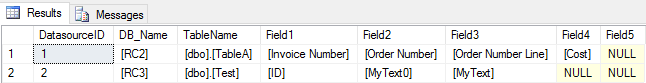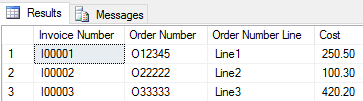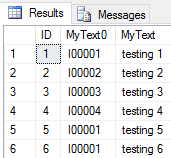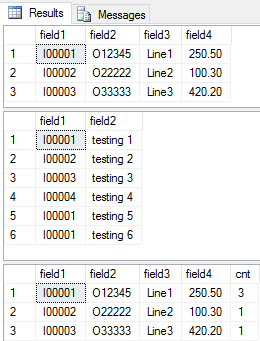# How to dynamically return a table structure using a Stored Procedure

(NIV) Hebrews 10:35 So do not throw away your confidence; it will be richly rewarded.
Published:
Updated:
A Stored Procedure in Microsoft SQL Server is a powerful feature that it can be used to execute the Data Manipulation Language (DML) or Data Definition Language (DDL).
Depending on business requirements, a single Stored Procedure can return different results based on different supplied parameters.

The Issue

I recently participated in this question about creating a Dynamic SQL script, and on review I found that a stored procedure will also do the trick.

The user has indicated that he has a table that stores the database objects name from a Source Table. That data will be used in order to generate a dynamic Select query.

Say we have the following data in the Source Table:Also say that the user is passing a DatasourceID with value of 1.

What we want is a simple Select query based on the DB_Name, Table_Name and Fields indicated in that particular record. In this case, the following query should be returned:

``````SELECT [Invoice Number], [Order Number], [Order Number Line], [Cost] FROM [RC2].[dbo].[TableA]
``````

This is similar for DatasourceID with value of 2, the following SQL statement will be returned:

``````SELECT [MyText0], [MyText] FROM [RC3].[dbo].[Test]
``````

Points to check

1. A Valid name for the database objects needs to exist as saved in the source table records.
2. Need to have read permissions to access to the database objects indicated in the source table records.

Proposed Resolution

The script posted should be workable for MSSQL 2008 R2 and above.

This can be resolved by creating a Stored Procedure which can dynamically return different table's data based on the passing in a different parameter. In order to do so, we would need to execute the following steps.

1. Create the database structure for source table

``````USE [RC]
GO

IF  EXISTS (SELECT * FROM sys.objects WHERE object_id = OBJECT_ID(N'[dbo].[SourceTable]') AND type in (N'U'))
DROP TABLE [dbo].[SourceTable]
GO

CREATE TABLE [dbo].[SourceTable](
[DatasourceID] [int] IDENTITY(1,1) NOT NULL,
[DB_Name] [varchar](20) NULL,
[TableName] [varchar](20) NULL,
[Field1] [varchar](20) NULL,
[Field2] [varchar](20) NULL,
[Field3] [varchar](20) NULL,
[Field4] [varchar](20) NULL,
[Field5] [varchar](20) NULL,
CONSTRAINT [PK_SourceTable] PRIMARY KEY CLUSTERED
(
[DatasourceID] ASC
)WITH (PAD_INDEX  = OFF, STATISTICS_NORECOMPUTE  = OFF, IGNORE_DUP_KEY = OFF, ALLOW_ROW_LOCKS  = ON, ALLOW_PAGE_LOCKS  = ON) ON [PRIMARY]
) ON [PRIMARY]

GO
``````

2. Create some test tables in different schemas

for example:

``````USE [RC2]
GO

IF  EXISTS (SELECT * FROM sys.objects WHERE object_id = OBJECT_ID(N'[dbo].[TableA]') AND type in (N'U'))
DROP TABLE [dbo].[TableA]
GO

CREATE TABLE [dbo].[TableA](
[Invoice Number] [varchar](50) NULL,
[Order Number] [varchar](50) NULL,
[Order Number Line] [varchar](50) NULL,
[Cost] [money] NULL
) ON [PRIMARY]

GO

USE [RC3]
GO

IF  EXISTS (SELECT * FROM sys.objects WHERE object_id = OBJECT_ID(N'[dbo].[Test]') AND type in (N'U'))
DROP TABLE [dbo].[Test]
GO

CREATE TABLE [dbo].[Test](
[ID] [int] IDENTITY(1,1) NOT NULL,
[MyText0] [varchar](50) NULL,
[MyText] [nvarchar](50) NULL,
CONSTRAINT [PK_Test] PRIMARY KEY CLUSTERED
(
[ID] ASC
)WITH (PAD_INDEX  = OFF, STATISTICS_NORECOMPUTE  = OFF, IGNORE_DUP_KEY = OFF, ALLOW_ROW_LOCKS  = ON, ALLOW_PAGE_LOCKS  = ON) ON [PRIMARY]
) ON [PRIMARY]

GO
``````

3. Insert some test data into source table

``````USE [RC]
GO

INSERT INTO SourceTable
([DB_Name], [TableName], [Field1], [Field2], [Field3], [Field4], [Field5]) VALUES
('[RC2]', '[dbo].[TableA]', '[Invoice Number]', '[Order Number]', '[Order Number Line]', '[Cost]', NULL),
('[RC3]', '[dbo].[Test]', '[MyText0]', '[MyText]', NULL, NULL, NULL);

GO

``````

4. Insert some test data of targeted tables

``````USE [RC2]
GO

INSERT INTO TableA
([Invoice Number], [Order Number], [Order Number Line], [Cost]) VALUES
('I00001', 'O12345', 'Line1', 250.50),
('I00002', 'O22222', 'Line2', 100.30),
('I00003', 'O33333', 'Line3', 420.20);

GO

USE [RC3]
GO

INSERT INTO Test
([MyText0], [MyText]) VALUES
('I00001', 'testing 1'),
('I00002', 'testing 2'),
('I00003', 'testing 3'),
('I00004', 'testing 4'),
('I00001', 'testing 5'),
('I00001', 'testing 6');

GO
``````

Now we have the following data in these tables in different schemas

``````SELECT * FROM [RC].[dbo].[SourceTable]
``````Figure 1 - Table Schema Results

``````SELECT * FROM [RC2].[dbo].[TableA]
``````Figure 2 - Sample Result Set

``````SELECT * FROM [RC3].[dbo].[Test]
``````Figure 3 - Sample Result Set

5. Create a Stored Procedure to accept a parameter and return the relevant data

``````USE [RC]
GO

IF  EXISTS (SELECT * FROM sys.objects WHERE object_id = OBJECT_ID(N'[dbo].[getTable]') AND type in (N'P', N'PC'))
DROP PROCEDURE [dbo].[getTable]
GO

CREATE PROCEDURE [dbo].[getTable]
@DatasourceID INT
AS
BEGIN
DECLARE
@DB_Name VARCHAR(20),
@TableName VARCHAR(20),
@Field1 VARCHAR(20),
@Field2 VARCHAR(20),
@Field3 VARCHAR(20),
@Field4 VARCHAR(20),
@Field5 VARCHAR(20),
@SQL NVARCHAR(500)

SET NOCOUNT ON

IF EXISTS(SELECT * FROM [SourceTable] WHERE DatasourceID = @DatasourceID)
BEGIN
SELECT
@DB_Name = [DB_Name],
@TableName = [TableName],
@Field1 = Field1,
@Field2 = Field2,
@Field3 = Field3,
@Field4 = Field4,
@Field5 = Field5
FROM [SourceTable] WHERE DatasourceID = @DatasourceID

SET @SQL = ''
IF  @Field1 IS NOT NULL
SET @SQL = @SQL + @Field1 + ', '
IF  @Field2 IS NOT NULL
SET @SQL = @SQL + @Field2 + ', '
IF  @Field3 IS NOT NULL
SET @SQL = @SQL + @Field3 + ', '
IF  @Field4 IS NOT NULL
SET @SQL = @SQL + @Field4 + ', '
IF  @Field5 IS NOT NULL
SET @SQL = @SQL + @Field5 + ', '

SET @SQL = 'SELECT ' + SUBSTRING(@SQL, 1, LEN(@SQL) - 1) +
' FROM ' + @DB_Name + '.' + @TableName
PRINT @SQL

EXEC sp_executesql @SQL
END
ELSE
PRINT 'No Record Matched'
END

GO
``````

6. Execute the Stored Procedure

Run the following SQL:

``````EXEC [RC].dbo.getTable 1
``````

The results below:Figure 4 - (this is exactly the same as what is returned by using a Select statement indicated in Figure 2 above)

``````EXEC [RC].dbo.getTable 2
``````

The results below:Figure 5 - (this is exactly the same as what is returned by using a Select statement as indicated in Image 3 above)

7. Join the results returned from the Stored Procedure with other tables

First we need to declare a table variable, we can also define a temporary table if necessary. In general, both ways work.

``````DECLARE @anotherTable1 TABLE
(
field1 NVARCHAR(MAX),
field2 NVARCHAR(MAX),
field3 NVARCHAR(MAX),
field4 NVARCHAR(MAX)
)
``````

When you know the exact data type of the expected result, you may change the NVARCHAR(MAX) to that expected data type.

Then Insert the results of the Stored Procedure into the table variable

``````INSERT INTO @anotherTable1
EXEC [RC].dbo.getTable 1
``````

Now do the same for another variable

``````DECLARE @anotherTable2 TABLE
(
field1 NVARCHAR(MAX),
field2 NVARCHAR(MAX)
)
INSERT INTO @anotherTable2
EXEC [RC].dbo.getTable 2
``````

8. The whole process

Now as a whole (as what indicated in Step 7), we do some checking and make sure these are working

``````DECLARE @anotherTable1 TABLE
(
field1 NVARCHAR(MAX),
field2 NVARCHAR(MAX),
field3 NVARCHAR(MAX),
field4 NVARCHAR(MAX)
)
INSERT INTO @anotherTable1
EXEC [RC].dbo.getTable 1

DECLARE @anotherTable2 TABLE
(
field1 NVARCHAR(MAX),
field2 NVARCHAR(MAX)
)
INSERT INTO @anotherTable2
EXEC [RC].dbo.getTable 2

SELECT * FROM @anotherTable1
SELECT * FROM @anotherTable2

SELECT a.field1, a.field2, a.field3, a.field4, count(b.field1) cnt
FROM @anotherTable1 a
INNER JOIN @anotherTable2 b
ON a.field1 = b.field1
GROUP BY a.field1, a.field2, a.field3, a.field4
``````

The results below:Figure 6 - Final Result Set

Some questions that may arise:

1. Can we use the same approach by using a Table-Valued Function instead?

2. Can we adding the Condition to this Stored Procedure?

I may write another article to address the questions mentioned above.

0
6,420 Views
(NIV) Hebrews 10:35 So do not throw away your confidence; it will be richly rewarded.